# hac

Heteroscedasticity and autocorrelation consistent covariance estimators

## Syntax

[EstCoeffCov,se,coeff] = hac(X,y)
[EstCoeffCov,se,coeff] = hac(Mdl)
[CovTbl,CoeffTbl] = hac(Tbl)
[___] = hac(___,Name=Value)

## Description

example

[EstCoeffCov,se,coeff] = hac(X,y) returns a robust covariance matrix estimate EstCoeffCov, and vectors of corrected standard errors se and OLS coefficient estimates coeff from applying ordinary least squares (OLS) on the multiple linear regression models y = Xβ + ε under general forms of heteroscedasticity and autocorrelation in the innovations process ε. y is a vector of response data and X is a matrix of predictor data.

example

[EstCoeffCov,se,coeff] = hac(Mdl) returns a robust covariance matrix estimate, and vectors of corrected standard errors and OLS coefficient estimates from a fitted multiple linear regression model Mdl, as returned by fitlm.

example

[CovTbl,CoeffTbl] = hac(Tbl) returns a robust covariance matrix estimate in the table CovTbl, and corrected standard errors and OLS coefficient estimates in the table CoeffTbl from a fitted multiple linear regression model of the variables in the table or timetable Tbl.The response variable in the regression is the last table variable, and all other variables are the predictor variables. To select a different response variable for the regression, use the ResponseVariable name-value argument. To select different predictor variables, use the PredictorNames name-value argument.

example

[___] = hac(___,Name=Value) specifies options using one or more name-value arguments in addition to any of the input argument combinations in previous syntaxes. hac returns the output argument combination for the corresponding input arguments.For example, hac(Tbl,ResponseVariable="GDP",Type="HC") provides a heteroscedasticity-consistent coefficient covariance estimate of a regression where the table variable GDP is the response while all other variables are predictors.

## Examples

collapse all

By default, hac returns the Newey-West coefficient covariance estimate, which is appropriate when residuals from a linear regression fit show evidence of heteroscedasticity and autocorrelation.

Simulate data from a linear model in which the innovations process is heteroscedastic and autocorrelated. Compare coefficient covariance estimates from regular OLS and the Newey-West estimator by using the default options of hac.

Simulate Data

Simulate a 1000-path series of data from the following innovations process.

$\begin{array}{l}{\epsilon }_{t}=0.5{\epsilon }_{t-1}+{u}_{t}\\ {u}_{t}\sim N\left(0,{\sigma }_{t}^{2}\right),\end{array}$

where ${\sigma }_{\mathit{t}}^{2}=0.001\mathit{t}$ and ${\epsilon }_{0}=0.1$.

rng(1); % For reproducibility T = 1000; sigma2 = 0.001*(1:T)'; ut = randn(T,1).*sqrt(sigma2); eMdl = arima(AR=0.5,Constant=0,Variance=1); et = filter(eMdl,ut,Y0=0.1); 

Simulate responses from the following linear model.

${y}_{t}={X}_{t}\left[\begin{array}{c}1\\ 2\\ 3\end{array}\right]+{\epsilon }_{t},$

where

$\begin{array}{l}{X}_{t}=\left[\begin{array}{ccc}1& {x}_{1,t}& {x}_{2,t}\end{array}\right]\\ {x}_{j,t}\sim N\left(j,\frac{j}{2}\right).\end{array}$

X = [1 2] + randn(T,2).*sqrt([1/2 1]); beta = (1:3)'; y = [ones(T,1) X]*beta + et;

Fit Linear Model

Fit a CLM to the simulated data. Plot the residuals in case order and plot each residual with respect to the previous residual.

Mdl = fitlm(X,y); tiledlayout(2,1) nexttile plotResiduals(Mdl,"caseorder") nexttile plotResiduals(Mdl,"lagged")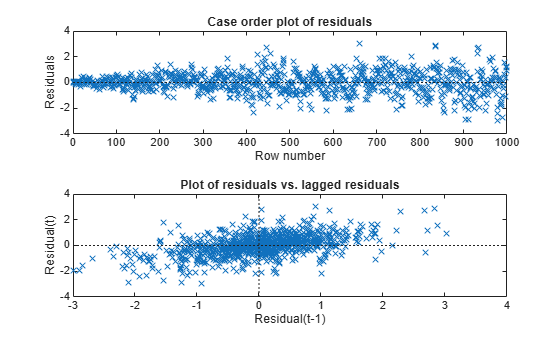The residuals exhibit heteroscedasticity and autocorrelation.

Compute Newey-West Coefficient Covariance Estimate

Estimate the Newey-West covariance, which accounts for the heteroscedasticity and autocorrelation of the residuals, by passing the data to hac. Return standard errors and coefficients. Compare results against the CLM approach.

[EstCoeffCov,se,coeff] = hac(X,y)
Estimator type: HAC Estimation method: BT Bandwidth: 11.1758 Whitening order: 0 Effective sample size: 1000 Small sample correction: on Coefficient Covariances: | Const x1 x2 ----------------------------------- Const | 0.0042 -0.0009 -0.0011 x1 | -0.0009 0.0012 -0.0000 x2 | -0.0011 -0.0000 0.0006 
EstCoeffCov = 3×3 0.0042 -0.0009 -0.0011 -0.0009 0.0012 -0.0000 -0.0011 -0.0000 0.0006 
se = 3×1 0.0645 0.0341 0.0254 
coeff = 3×1 1.0444 2.0153 2.9567 
EstCoeffCovOLS = Mdl.CoefficientCovariance
EstCoeffCovOLS = 3×3 0.0045 -0.0012 -0.0013 -0.0012 0.0012 -0.0000 -0.0013 -0.0000 0.0007 
seOLS = Mdl.Coefficients.SE
seOLS = 3×1 0.0669 0.0348 0.0257 
coeffOLS = Mdl.Coefficients.Estimate
coeffOLS = 3×1 1.0444 2.0153 2.9567 

Between the CLM and Newey-West approaches, the coefficient estimates are equal, but the standard errors and covariances are different.

Model an automobile's price with its curb weight, engine size, and cylinder bore diameter using the linear model:

${\text{price}}_{i}={\beta }_{0}+{\beta }_{1}{\text{curbWeight}}_{i}+{\beta }_{2}{\text{engineSize}}_{i}+{\beta }_{3}{\text{bore}}_{i}+{\epsilon }_{i}.$

Estimate model coefficients and White's robust covariance.

Load the 1985 automobile imports data set import-85.mat, which contains all variables in the matrix X. Collect the variables in the regression model in a table.

load imports-85 varnames = ["CurbWeight" "EngineSize" "Bore" "Price"]; Tbl = array2table(X(:,[7:9 15]),VariableNames=varnames);

Fit the linear model to the data and plot the residuals versus the fitted values.

Mdl = fitlm(Tbl); plotResiduals(Mdl,"fitted")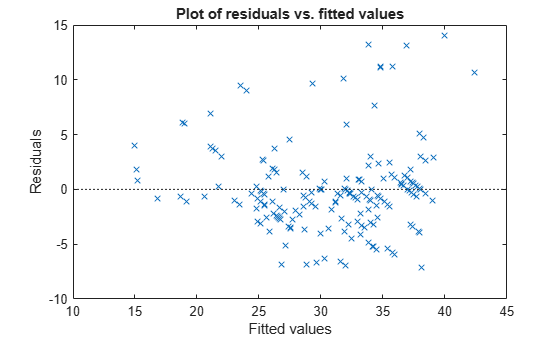The residuals appear to flare out, which indicates heteroscedasticity.

Use hac to compute the usual OLS coefficient covariance estimate and White's robust covariance estimate.

[LSCov,lsSE,lsCoeff] = hac(Mdl,Type="HC",Weights="CLM", ... Display="off") % OLS estimates
LSCov = 4×4 13.7124 0.0000 0.0120 -4.5609 0.0000 0.0000 -0.0000 -0.0005 0.0120 -0.0000 0.0002 -0.0017 -4.5609 -0.0005 -0.0017 1.8195 
lsSE = 4×1 3.7030 0.0011 0.0133 1.3489 
lsCoeff = 4×1 64.0948 -0.0087 -0.0158 -2.6998 
[WhiteCov,whiteSE,whiteCoeff] = hac(Mdl,Type="HC",Weights="HC0", ... Display="off") % White's estimates
WhiteCov = 4×4 15.5122 -0.0008 0.0137 -4.4461 -0.0008 0.0000 -0.0000 -0.0003 0.0137 -0.0000 0.0001 -0.0010 -4.4461 -0.0003 -0.0010 1.5707 
whiteSE = 4×1 3.9385 0.0011 0.0105 1.2533 
whiteCoeff = 4×1 64.0948 -0.0087 -0.0158 -2.6998 

The OLS coefficient covariance estimate is not equal to White's robust estimate because the latter accounts for the heteroscedasticity in the residuals.

Model the nominal GNP (GNPN) with consumer price index (CPI), real wages (WR), and the money stock (MS) using the linear model:

${\text{GNPN}}_{i}={\beta }_{0}+{\beta }_{1}{\text{CPI}}_{i}+{\beta }_{2}{\text{WR}}_{i}+{\beta }_{3}{\text{MS}}_{i}+{\epsilon }_{i}.$

Estimate the model coefficients and the Newey-West OLS coefficient covariance matrix.

Load the Nelson Plosser data set Data_NelsonPlosser.mat, which contains the table DataTable. Remove all observations containing at least one NaN and compute the log of the series.

load Data_NelsonPlosser Tbl = rmmissing(DataTable); LogTbl = varfun(@log,Tbl); T = height(LogTbl);

Fit the linear model using the log of all series. Plot the residuals by case order and plot a correlogram of them.

Mdl = fitlm(LogTbl,"log_GNPN ~ 1 + log_CPI + log_WR + log_MS"); resid = Mdl.Residuals.Raw; figure tiledlayout(2,1) nexttile plotResiduals(Mdl,"caseorder") axis tight nexttile autocorr(resid)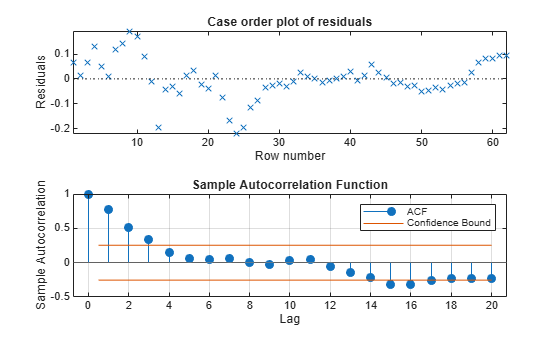The residual plot exhibits signs of heteroscedasticity, autocorrelation, and possibly model misspecification. The sample autocorrelation function clearly exhibits autocorrelation.

Calculate the lag selection parameter for the standard Newey-West HAC estimate .

maxLag = floor(4*(T/100)^(2/9));

Estimate the standard Newey-West OLS coefficient covariance by using hac. Set the bandwidth to maxLag + 1. Display the OLS coefficient estimates, and their standard errors and covariance matrix.

prednames = ["log_CPI" "log_WR" "log_MS"]; [CovTbl,CoeffTbl] = hac(LogTbl,ResponseVariable="log_GNPN", ... PredictorVariables=prednames,Bandwidth=maxLag + 1, ... Display="full")
Estimator type: HAC Estimation method: BT Bandwidth: 4.0000 Whitening order: 0 Effective sample size: 62 Small sample correction: on Coefficient Estimates: | Coeff SE --------------------------- Const | -4.3473 0.4297 log_CPI | 0.9947 0.1001 log_WR | 1.3954 0.1283 log_MS | 0.0789 0.0625 Coefficient Covariances: | Const log_CPI log_WR log_MS ---------------------------------------------- Const | 0.1847 -0.0318 -0.0433 0.0239 log_CPI | -0.0318 0.0100 0.0027 -0.0043 log_WR | -0.0433 0.0027 0.0165 -0.0064 log_MS | 0.0239 -0.0043 -0.0064 0.0039 
CovTbl=4×4 table Const log_CPI log_WR log_MS _________ __________ _________ __________ Const 0.18468 -0.03177 -0.043323 0.023939 log_CPI -0.03177 0.010015 0.0026864 -0.0043025 log_WR -0.043323 0.0026864 0.016454 -0.006435 log_MS 0.023939 -0.0043025 -0.006435 0.0039086 
CoeffTbl=4×2 table Coeff SE ________ ________ Const -4.3473 0.42974 log_CPI 0.99472 0.10008 log_WR 1.3954 0.12827 log_MS 0.078933 0.062519 

The first table in the output contains the OLS estimates (${\underset{}{\overset{ˆ}{\beta }}}_{0},...,{\underset{}{\overset{ˆ}{\beta }}}_{3}$, respectively) and their standard errors. The second table is the Newey-West coefficient covariance matrix estimate. For example, $Cov\left({\underset{}{\overset{ˆ}{\beta }}}_{1},{\underset{}{\overset{ˆ}{\beta }}}_{2}\right)=0.0027$. hac returns tables of estimates when you supply a table of data.

Plot the kernel density functions available in hac.

Set domain, x, and range w.

x = (0:0.001:3.2)'; w = zeros(size(x));

Compute the truncated kernel density.

cTR = 2; % Renormalization constant TR = (abs(x) <= 1); TRRn = (abs(cTR*x) <= 1); wTR = w; wTR(TR) = 1; wTRRn = w; wTRRn(TRRn) = 1;

Compute the Bartlett kernel density.

cBT = 2/3; % Renormalization constant BT = (abs(x) <= 1); BTRn = (abs(cBT*x) <= 1); wBT = w; wBT(BT) = 1-abs(x(BT)); wBTRn = w; wBTRn(BTRn) = 1-abs(cBT*x(BTRn));

Compute the Parzen kernel density.

cPZ = 0.539285; % Renormalization constant PZ1 = (abs(x) >= 0) & (abs(x) <= 1/2); PZ2 = (abs(x) >= 1/2) & (abs(x) <= 1); PZ1Rn = (abs(cPZ*x) >= 0) & (abs(cPZ*x) <= 1/2); PZ2Rn = (abs(cPZ*x) >= 1/2) & (abs(cPZ*x) <= 1); wPZ = w; wPZ(PZ1) = 1-6*x(PZ1).^2+6*abs(x(PZ1)).^3; wPZ(PZ2) = 2*(1-abs(x(PZ2))).^3; wPZRn = w; wPZRn(PZ1Rn) = 1-6*(cPZ*x(PZ1Rn)).^2 ... + 6*abs(cPZ*x(PZ1Rn)).^3; wPZRn(PZ2Rn) = 2*(1-abs(cPZ*x(PZ2Rn))).^3;

Compute the Tukey-Hanning kernel density.

cTH = 3/4; % Renormalization constant TH = (abs(x) <= 1); THRn = (abs(cTH*x) <= 1); wTH = w; wTH(TH) = (1+cos(pi*x(TH)))/2; wTHRn = w; wTHRn(THRn) = (1+cos(pi*cTH*x(THRn)))/2;

Compute the quadratic spectral kernel density.

argQS = 6*pi*x/5; w1 = 3./(argQS.^2); w2 = (sin(argQS)./argQS)-cos(argQS); wQS = w1.*w2; wQS(x == 0) = 1; wQSRn = wQS; % Renormalization constant = 1

Plot the kernel densities.

figure plot(x,[wTR wBT wPZ wTH wQS],LineWidth=2) hold on plot(x,w,"k",LineWidth=2) axis([0 3.2 -0.2 1.2]) grid on title("\bf HAC Kernels") legend(["Truncated" "Bartlett" "Parzen" "Tukey-Hanning", ... "Quadratic spectral"]) xlabel("Covariance Lag") ylabel("Weight")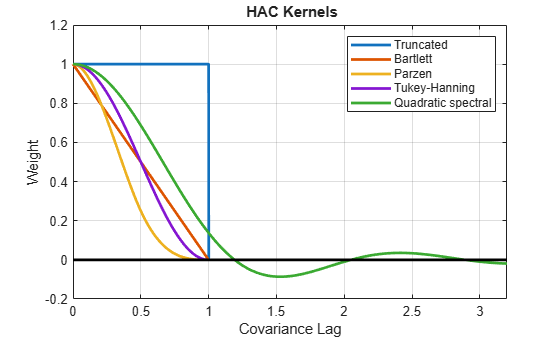All graphs are truncated at Covariance Lag = 1, except for the quadratic spectral. The quadratic spectral density approaches 0 as Covariance Lag gets large, but does not get truncated.

Plot renormalized kernels. Unlike the densities in the previous plot, these have the same asymptotic variance .

figure plot(x,[wTRRn,wBTRn,wPZRn,wTHRn,wQSRn],LineWidth=2) hold on plot(x,w,"k",LineWidth=2) axis([0 3.2 -0.2 1.2]) grid on title("{\bf Renormalized HAC Kernels} (Equal Asymptotic Variance)") legend(["Truncated" "Bartlett" "Parzen" "Tukey-Hanning" "Quadratic spectral"]) xlabel("Covariance Lag") ylabel("Weight")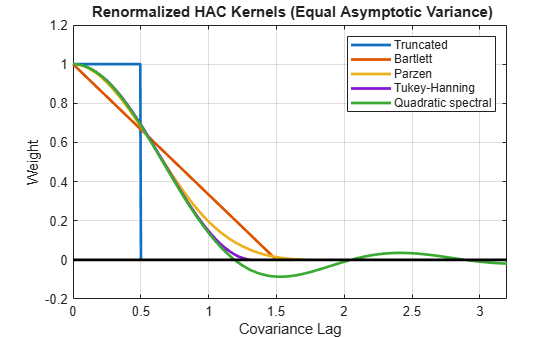Examine the effects of changing the bandwidth parameter on the quadratic spectral density.

Assign several bandwidth values to b. Assign the domain to l. Calculate x = l/|b|.

b = (1:5)'; l = (0:0.1:10); x = bsxfun(@rdivide,repmat(l,[size(b) 1]),b)';

Calculate the quadratic spectral density under the domain for each bandwidth value.

argQS = 6*pi*x/5; w1 = 3./(argQS.^2); w2 = (sin(argQS)./argQS)-cos(argQS); wQS = w1.*w2; wQS(x == 0) = 1;

figure plot(l,wQS,LineWidth=2); grid on xlabel("Covariance Lag"); ylabel("Quadratic Spectral Density"); title("\bf Change in Bandwidth for Quadratic Spectral Denisty"); legend("Bandwidth = 1","Bandwidth = 2","Bandwidth = 3", ... "Bandwidth = 4","Bandwidth = 5");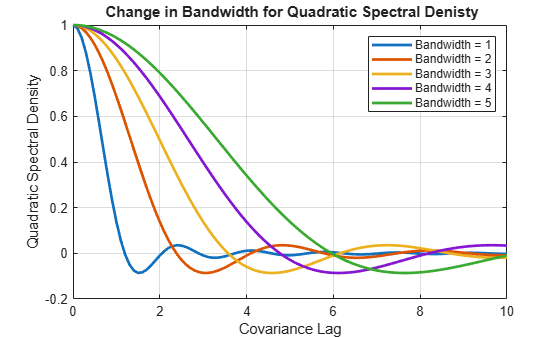As the bandwidth increases, the kernel imparts more weight to larger lags.

## Input Arguments

collapse all

Predictor data X for the multiple linear regression model, specified as a numObs-by-numPreds numeric matrix.

Each row represents one of the numObs observations and each column represents one of the numPreds predictor variables.

Data Types: double

Response data y for the multiple linear regression model, specified as a numObs-by-1 numeric vector. Rows of y and X correspond.

Data Types: double

Fitted linear model, specified as a LinearModel object returned by fitlm.

Combined predictor and response data for the multiple linear regression model, specified as a table or timetable with numObs rows. Each row of Tbl is an observation.

The test regresses the response variable, which is the last variable in Tbl, on the predictor variables, which are all other variables in Tbl. To select a different response variable for the regression, use the ResponseVariable name-value argument. To select different predictor variables, use the PredictorNames name-value argument to select numPreds predictors.

Note

NaNs in X, y, or Tbl indicate missing values, and hac removes observations containing at least one NaN. That is, to remove NaNs in X or y, hac merges the variables [X y], and then it uses list-wise deletion to remove any row that contains at least one NaN. hac also removes any row of Tbl containing at least one NaN. Removing NaNs in the data reduces the sample size and can create irregular time series.

### Name-Value Arguments

Specify optional pairs of arguments as Name1=Value1,...,NameN=ValueN, where Name is the argument name and Value is the corresponding value. Name-value arguments must appear after other arguments, but the order of the pairs does not matter.

Before R2021a, use commas to separate each name and value, and enclose Name in quotes.

Example: hac(Tbl,ResponseVariable="GDP",Type="HC") provides a heteroscedasticity-consistent coefficient covariance estimate of a regression where the table variable GDP is the response while all other variables are predictors.

Unique variable names used in displays and plots of the results, specified as a string vector or cell vector of strings of a length numCoeffs.

VarNames must include names for all coefficients in the model.

• If you do not supply an input model Mdl:

• If Intercept=true, VarNames(1) is the name of the intercept, and VarNames(j + 1) specifies the name to use for variable X(:,j) or PredictorVariables(j).

• If Intercept=false, VarNames(j) specifies the name to use for variable X(:,j) or PredictorVariables(j).

• If you supply an input model Mdl, you must additionally supply names for higher-order terms (for example, "x1^2" or "x1:x2").

The default is one of the following alternatives with 'Const' representing the intercept term when it is present:

• {'x1' 'x2' ...} when you supply inputs X and y

• Tbl.Properties.VariableNames when you supply input table or timetable Tbl

• Mdl.CoefficientNames when you supply the input LinearModel object Mdl.

Example: VarNames=["Const" "AGE" "BBD"]

Data Types: char | cell | string

Flag to include a model intercept, specified as a value in this table.

ValueDescription
truehac include an intercept in the model.
falsehac exclude an intercept from the model.

The number of model coefficients numCoeffs is numPreds + Intercept.

If you specify Mdl, hac ignores Intercept and uses the intercept in Mdl.

Example: Intercept=false

Data Types: logical

Coefficient covariance estimator type, specified as a value in this table.

ValueCovariance EstimateUsage
"HAC"Heteroscedasticity-and-autocorrelation-consistent (HAC) estimate as described in , , , and When residuals exhibit both heteroscedasticity and autocorrelation
"HC"Heteroscedasticity-consistent (HC) estimate as described in , , and When residuals exhibit only heteroscedasticity

Example: Type="HC"

Data Types: char

Coefficient covariance estimator weighting scheme, specified as a value in the following tables or a length numObs numeric vector.

Set Weights to specify the structure of the innovations covariance Ω. hac uses this specification to compute $\stackrel{^}{\Phi }={X}^{\prime }\stackrel{^}{\Omega }X$ (see Sandwich Estimators).

• If Type="HC", then $\stackrel{^}{\Omega }=diag\left(\omega \right),$ where ωi estimates innovation variance i, i = 1,...,T, and T = numObs. hac estimates ωi using the residual i, εi, its leverage ${h}_{i}={x}_{i}{\left({X}^{\prime }X\right)}^{-1}{x}_{i}^{\prime },$ ${d}_{i}=\mathrm{min}\left(4,\frac{{h}_{i}}{\overline{h}}\right)$, and the degrees of freedom, dfe.

ValueWeightReference
"CLM"

${\omega }_{i}=\frac{1}{dfe}\sum _{i=1}^{T}{\epsilon }_{i}^{2}$


"HCO" (default when Type="HC")

${\omega }_{i}={\epsilon }_{i}^{2}$


"HC1"

${\omega }_{i}=\frac{T}{dfe}{\epsilon }_{i}^{2}$


"HC2"

${\omega }_{i}=\frac{{\epsilon }_{i}^{2}}{1-{h}_{i}}$


"HC3"

${\omega }_{i}=\frac{{\epsilon }_{i}^{2}}{{\left(1-{h}_{i}\right)}^{2}}$


"HC4"

${\omega }_{i}=\frac{{\epsilon }_{i}^{2}}{{\left(1-{h}_{i}\right)}^{{d}_{i}}}$



• If Type="HAC", hac weights the component products that form $\stackrel{^}{\Phi }$, ${x}_{i}{}^{\prime }{\epsilon }_{i}{\epsilon }_{j}{x}_{j}$, using a measure of autocorrelation strength, ω(l), at each lag, l = |ij|. ω(l) = k(l/b), where k is a kernel density estimator and b is a bandwidth specified by the Bandwidth name-value argument.

ValueKernel DensityKernel Density FunctionReference
"TR"

Truncated

This setting produces the White estimator in .
"BT" (default when Type="HAC")

Bartlett

This setting produces the Newey-West estimator in .
"PZ"

Parzen

This setting produces the Gallant estimator in .
"TH"

Tukey-Hanning


"QS"

$k\left(z\right)=\frac{25}{12{\pi }^{2}{z}^{2}}\left(\frac{\mathrm{sin}\left(6\pi z/5\right)}{6\pi z/5}-\mathrm{cos}\left(6\pi z/5\right)\right)$



For a visual description of the kernel densities, see Plot Kernel Densities.

• For either value of Type, you can set Weights to any length numObs numeric vector without containing NaNs. However, such a custom vector might not produce positive definite matrices.

If you set Weights to a numeric vector, hac concatenates the input data and the weights, and removes all rows containing at least one NaN.

Example: Weights="QS"

Data Types: double | char | string

Bandwidth value or method name indicating how hac estimates the data-driven bandwidth parameter, specified as a positive scalar or a method name.

• If Type="HC", hac ignores Bandwidth.

• If Type="HAC", you must provide a nonzero scalar for the bandwidth or use a name in the following table to indicate which model and method hac uses to estimate the data-driven bandwidth. For details, see .

Method NameModelMethod
"AR1"AR(1)Maximum likelihood
"AR1MLE"AR(1)Maximum likelihood
"AR1OLS"AR(1)OLS
"ARMA11"ARMA(1,1)Maximum likelihood

Example: Bandwidth=floor(4*(T/100)^(2/9))+1

Data Types: double | char

Flag to apply the small sample correction to the estimated covariance matrix, specified as a logical value.

The small sample correction factor is $\frac{T}{dfe}$, where T is the sample size and dfe is the residual degrees of freedom. For details, see .

ValueDescription
truehac applies the small sample correction.
falsehac do not apply the small sample correction.

The defaults is:

• false when Type="HC"

• true when Type="HAC"

Example: SmallT=false

Data Types: logical

Lag order for the VAR model prewhitening filter, specified as a nonnegative integer.

For details on prewhitening filters, see .

• If Type="HC", hac ignores Whiten.

• If Whiten=0, hac does not apply a prewhitening filter.

Example: Whiten=1

Data Types: double

Command window display control, specified as a value in this table. hac displays results in tabular form.

ValueDescription
"cov"hac displays a table of the estimated covariances of the OLS coefficients.
"full"hac displays a table of coefficient estimates, their standard errors, and their estimated covariances.
"off"hac do not display an estimates table to the Command Window.

Example: Display="off"

Variable in Tbl to use for response, specified as a string vector or cell vector of character vectors containing variable names in Tbl.Properties.VariableNames, or an integer or logical vector representing the indices of names. The selected variables must be numeric.

hac uses the same specified response variable for all tests.

Example: ResponseVariable="GDP"

Example: ResponseVariable=[true false false false] or ResponseVariable=1 selects the first table variable as the response.

Data Types: double | logical | char | cell | string

Variables in Tbl to use for the predictors, specified as a string vector or cell vector of character vectors containing variable names in Tbl.Properties.VariableNames, or an integer or logical vector representing the indices of names. The selected variables must be numeric.

hac uses the same specified predictors for all tests.

By default, hac uses all variables in Tbl that are not specified by the ResponseVariable name-value argument.

Example: PredictorVariables=["UN" "CPI"]

Example: PredictorVariables=[false true true false] or DataVariables=[2 3] selects the second and third table variables.

Data Types: double | logical | char | cell | string

## Output Arguments

collapse all

Coefficient covariance estimate, returned as a numPreds-by-numPreds numeric matrix. hac returns EstCoeffCov when you supply the inputs X and y or Mdl.

• If you specify inputs X and y, rows and columns of EstCoeffCov correspond to the predictor matrix columns, with the first row and column corresponding to the intercept when Intercept=true. For example, in a model with an intercept, the estimated covariance of ${\stackrel{^}{\beta }}_{1}$ (corresponding to the predictor x1) and ${\stackrel{^}{\beta }}_{2}$ (corresponding to the predictor x2) are in positions (2,3) and (3,2) of EstCoeffCov, respectively.

• If you specify the input Mdl, rows and columns of EstCoeffCov correspond to Mdl.Coefficients.

Coefficient standard error estimates, returned as a length numPreds numeric vector whose elements are sqrt(diag(EstCoeffCov)). hac returns se when you supply the inputs X and y or Mdl.

• If you specify inputs X and y, rows of se correspond to the predictor matrix columns, with the first row corresponding to the intercept when Intercept=true. For example, in a model with an intercept, the estimated standard error of ${\stackrel{^}{\beta }}_{1}$ (corresponding to the predictor x1) is in position 2 of se, and is the square root of the value in position (2,2) of EstCoeffCov.

• If you specify the input Mdl, rows of se correspond to Mdl.Coefficients.

OLS coefficient estimates, returned as a numPreds numeric vector. hac returns coeff when you supply the inputs X and y or Mdl.

• If you specify inputs X and y, rows of coeff correspond to the predictor matrix columns, with the first row corresponding to the intercept when Intercept=true. For example, in a model with an intercept, the value of ${\stackrel{^}{\beta }}_{1}$ (corresponding to the predictor x1) is in position 2 of coeff.

• If you specify the input Mdl, rows of coeff correspond to Mdl.Coefficients.

Coefficient covariance matrix estimate, returned as a numCoeffs-by-numCoeffs table containing the coefficient covariance matrix estimate EstCoeffCov. hac returns CovTbl when you supply the input Tbl.

For each pair (i,j), CovTbl(i,j) contains the covariance estimate of coefficients i and j in the regression model. The label of row and variable j is VarNames(j), j = 1,…,numCoeffs.

Coefficient estimates and standard errors, returned as a numCoeffs-by-2 table. hac returns CoeffTbl when you supply the input Tbl.

For j = 1,…,numCoeffs, row j of CoeffTbl contains estimates of coefficient j in the regression model and it has label VarNames(j). The first variable Coeff contains the coefficient estimates coeff and the second variable SE contains the standard errors se.

collapse all

### Sandwich Estimators

This estimator has the form ${A}^{-1}B{A}^{-1}$.

The estimated covariance matrix that hac returns is called a sandwich estimator because of its form:

$c{\left({X}^{\prime }X\right)}^{-1}\stackrel{^}{\Phi }{\left({X}^{\prime }X\right)}^{-1},$

where ${\left({X}^{\prime }X\right)}^{-1}$ is the bread, $\stackrel{^}{\Phi }={X}^{\prime }\stackrel{^}{\Omega }X$ is the filling, and c is an optional small sample correction.

### Lag-Truncation Parameter

This parameter directs a kernel density to assign no weight to all lags above its value.

For kernel densities with unit-interval support, the bandwidth parameter, b, is often called the lag-truncation parameter since w(l) = k(l/b) = 0 for lags l > b.

## Tips

• To reduce bias in HAC estimators, prewhiten the input series . The prewhitening procedure tends to increase estimator variance and mean-squared error, but it can improve confidence interval coverage probabilities and reduce the over-rejection of t statistics.

## Algorithms

• The original White HC estimator, specified by the settings Type="HC",Weights="HC0" is justified asymptotically. The other values of the Weights name-value argument, "HC1", "HC2", "HC3", and "HC4", are meant to improve small-sample performance. Specify "HC3" or "HC4" in the presence of influential observations (see  and ).

• HAC estimators formed using the truncated kernel might not be positive semidefinite in finite samples.  proposes using the Bartlett kernel as a remedy, but the resulting estimator is suboptimal in terms of its rate of consistency. The quadratic spectral kernel achieves an optimal rate of consistency.

• The default estimation method for HAC bandwidth selection, specified by the Bandwidth name-value argument, is "AR1MLE", which is generally more accurate, but slower, than the AR(1) alternative "AR1OLS". If you specify Bandwidth="ARMA11", hac fits the model using maximum likelihood.

• Bandwidth selection models might exhibit sensitivity to the relative scale of the input predictors.

 Andrews, D. W. K. "Heteroskedasticity and Autocorrelation Consistent Covariance Matrix Estimation." Econometrica. Vol. 59, 1991, pp. 817–858.

 Andrews, D. W. K., and J. C. Monohan. "An Improved Heteroskedasticity and Autocorrelation Consistent Covariance Matrix Estimator." Econometrica. Vol. 60, 1992, pp. 953–966.

 Cribari-Neto, F. "Asymptotic Inference Under Heteroskedasticity of Unknown Form." Computational Statistics & Data Analysis. Vol. 45, 2004, pp. 215–233.

 den Haan, W. J., and A. Levin. "A Practitioner's Guide to Robust Covariance Matrix Estimation." In Handbook of Statistics. Edited by G. S. Maddala and C. R. Rao. Amsterdam: Elsevier, 1997.

 Frank, A., and A. Asuncion. UCI Machine Learning Repository. Irvine, CA: University of California, School of Information and Computer Science. https://archive.ics.uci.edu/ml/index.php, 2012.

 Gallant, A. R. Nonlinear Statistical Models. Hoboken, NJ: John Wiley & Sons, Inc., 1987.

 Kutner, M. H., C. J. Nachtsheim, J. Neter, and W. Li. Applied Linear Statistical Models. 5th ed. New York: McGraw-Hill/Irwin, 2005.

 Long, J. S., and L. H. Ervin. "Using Heteroscedasticity-Consistent Standard Errors in the Linear Regression Model." The American Statistician. Vol. 54, 2000, pp. 217–224.

 MacKinnon, J. G. "Numerical Distribution Functions for Unit Root and Cointegration Tests." Journal of Applied Econometrics. Vol. 11, 1996, pp. 601–618.

 Newey, W. K., and K. D. West. "A Simple, Positive Semidefinite, Heteroskedasticity and Autocorrelation Consistent Covariance Matrix." Econometrica. Vol. 55, 1987, pp. 703–708.

 Newey, W. K, and K. D. West. “Automatic Lag Selection in Covariance Matrix Estimation.” The Review of Economic Studies. Vol. 61 No. 4, 1994, pp. 631–653.

 White, H. "A Heteroskedasticity-Consistent Covariance Matrix and a Direct Test for Heteroskedasticity." Econometrica. Vol. 48, 1980, pp. 817–838.

 White, H. Asymptotic Theory for Econometricians. New York: Academic Press, 1984.